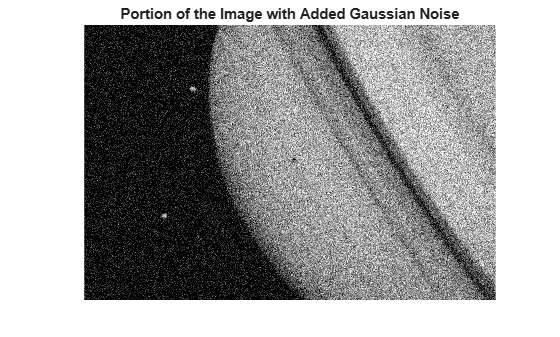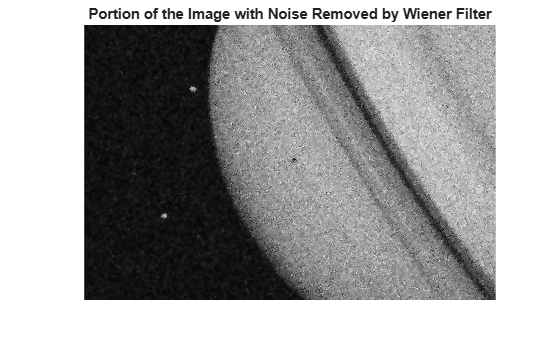# wiener2

2-D adaptive noise-removal filtering

The syntax `wiener2(I,[m n],[mblock nblock],noise)` has been removed. Use the `wiener2(I,[m n],noise)` syntax instead.

## Syntax

``J = wiener2(I,[m n],noise)``
``[J,noise_out] = wiener2(I,[m n])``

## Description

````J = wiener2(I,[m n],noise)` filters the grayscale image `I` using a pixel-wise adaptive low-pass Wiener filter. `[m n]` specifies the size (`m`-by-`n`) of the neighborhood used to estimate the local image mean and standard deviation. The additive noise (Gaussian white noise) power is assumed to be `noise`.The input image has been degraded by constant power additive noise. `wiener2` uses a pixelwise adaptive Wiener method based on statistics estimated from a local neighborhood of each pixel.```

example

````[J,noise_out] = wiener2(I,[m n])` returns the estimates of the additive noise power `wiener2` calculates before doing the filtering.```

## Examples

collapse all

This example shows how to use the `wiener2` function to apply a Wiener filter (a type of linear filter) to an image adaptively. The Wiener filter tailors itself to the local image variance. Where the variance is large, `wiener2` performs little smoothing. Where the variance is small, `wiener2` performs more smoothing.

This approach often produces better results than linear filtering. The adaptive filter is more selective than a comparable linear filter, preserving edges and other high-frequency parts of an image. In addition, there are no design tasks; the `wiener2` function handles all preliminary computations and implements the filter for an input image. `wiener2`, however, does require more computation time than linear filtering.

`wiener2` works best when the noise is constant-power ("white") additive noise, such as Gaussian noise. The example below applies `wiener2` to an image of Saturn with added Gaussian noise.

Read the image into the workspace.

`RGB = imread('saturn.png');`

Convert the image from truecolor to grayscale.

`I = im2gray(RGB);`

Add Gaussian noise to the image

`J = imnoise(I,'gaussian',0,0.025);`

Display the noisy image. Because the image is quite large, display only a portion of the image.

```imshow(J(600:1000,1:600)); title('Portion of the Image with Added Gaussian Noise');```Remove the noise using the `wiener2` function.

`K = wiener2(J,[5 5]);`

Display the processed image. Because the image is quite large, display only a portion of the image.

```figure imshow(K(600:1000,1:600)); title('Portion of the Image with Noise Removed by Wiener Filter');```## Input Arguments

collapse all

Input image, specified as a 2-D numeric array.

Data Types: `single` | `double` | `int16` | `uint8` | `uint16`

Neighborhood size, specified as a 2-element vector of the form `[m n]` where `m` is the number of rows and `n` is the number of columns.

Data Types: `single` | `double` | `int8` | `int16` | `int32` | `int64` | `uint8` | `uint16` | `uint32` | `uint64` | `logical`

Additive noise, specified as a numeric array. If you do not specify noise, then `wiener2` uses the mean of the local variance, `mean2(localVar)`.

Data Types: `single` | `double`

## Output Arguments

collapse all

Filtered image, returned as a numeric array of the same size and data type as the input image `I`.

Estimate of additive noise power, returned as a numeric array.

## Algorithms

`wiener2` estimates the local mean and variance around each pixel.

`$\mu =\frac{1}{NM}\sum _{{n}_{1},{n}_{2}\in \eta }a\left({n}_{1},{n}_{2}\right)$`

and

`${\sigma }^{2}=\frac{1}{NM}\sum _{{n}_{1},{n}_{2}\in \eta }{a}^{2}\left({n}_{1},{n}_{2}\right)-{\mu }^{2},$`

where $\eta$ is the N-by-M local neighborhood of each pixel in the image `A`. `wiener2` then creates a pixelwise Wiener filter using these estimates,

`$b\left({n}_{1},{n}_{2}\right)=\mu +\frac{{\sigma }^{2}-{\nu }^{2}}{{\sigma }^{2}}\left(a\left({n}_{1},{n}_{2}\right)-\mu \right),$`

where ν2 is the noise variance. If the noise variance is not given, `wiener2` uses the average of all the local estimated variances.

 Lim, Jae S. Two-Dimensional Signal and Image Processing, Englewood Cliffs, NJ, Prentice Hall, 1990, p. 548, equations 9.44, 9.45, and 9.46.